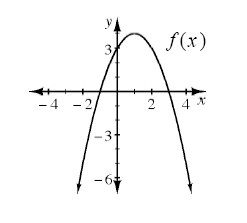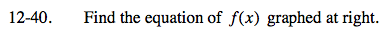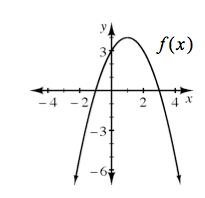### Home > CAAC > Chapter 12 > Lesson 12.2.1 > Problem12-40

12-40.

Find the equation of f(x) graphed below. Homework Help ✎Identify all of the intercepts.

Write the equation as a factored quadratic since the two values of x are known.

y = (x - 3)(x + 1)

Make the equation negative because the parabola opens downward.

y = -(x - 3)(x + 1)

Use a generic rectangle to multiply the factors together. Distribute the negative.